1800-1023-196

+91-120-4616500

# Application of Radioactivity

## Application of Radioactivity

Radioisotopes find numerous applications in a variety of areas such as medicine, agriculture, biology, chemistry, archeology, engineering and industry.

(1) Age determination: The age of earth has been determined by uranium dating technique as follows. Samples of uranium ores are found to contain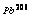as a result of long series of a- and b-decays. Now if it is assumed that the ore sample contained no lead at the moment of its formation, and if none of the lead formed from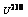decay has been lost then the measurement of the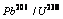ratio will give the value of time t of the mineral.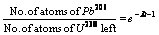where l is the decay constant of uranium-238

Alternatively,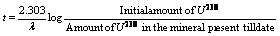Similarly, the less abundant isotope of uranium,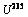eventually decays to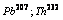decays to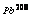and thus the ratios of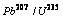andcan be used to determine the age of rocks and minerals.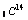(half-life 5760 years) was used by Willard Libby (Nobel lauret) in determining the age of carbon-bearing materials (e.g. wood, animal fossils, etc.) Carbon-14 is produced by the bombardment of nitrogen atoms present in the upper atmosphere with neutrons (from cosmic rays).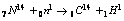Thus carbon-14 is oxidised to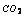and eventually ingested by plants and animals. The death of plants or animals puts an end to the intake of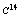from the atmosphere. After this the amount ofin the dead tissues starts decreasing due to its disintegration.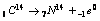It has been observed that on an average, one gram of radioactive carbon emits about 12 b-particles per minute. Thus by knowing either the amount of C-14 or the number of b-particles emitted per minute per gram of carbon at the initial and final (present) stages, the age of carbon material can be determined by using the following formulae.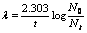or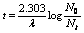where t = Age of the fossil, l = Decay constant,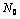= Initial radioactivity (in the fresh wood),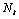= Radioactivity in the fossil

The above formula can be modified as,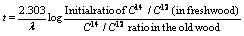Similarly, tritium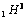has been used for dating purposes.

## NEET & AIIMS Exam Sample Papers

 AIIMS SAMPLE PAPERS View More NEET SAMPLE PAPERS View More